Function Repository Resource:

# InvoluteCurve

Compute the involute of a curve

Contributed by: Wolfram Staff (original content by Alfred Gray)
 ResourceFunction["InvoluteCurve"][c,t] computes the involute of a curve c with parameter t. ResourceFunction["InvoluteCurve"][c,{t,tf}] computes the involute by switching to numerical integration from 0 to tf.

## Details

An involute can be constructed by moving the end of a taut cord unwound from the contour of a planar curve. The locus of points traced out by the end of the cord is called the involute. Tautness means the cord is always tangent to the curve from which it is unwound.

## Examples

### Basic Examples (2)

Compute the involute of a circle:

 In:=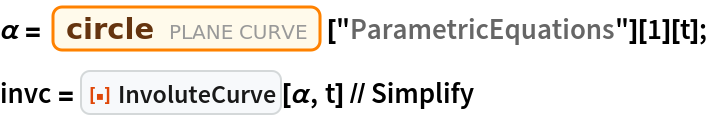Out=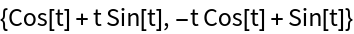Plot the circle involute and tangent lines along the circle:

 In:=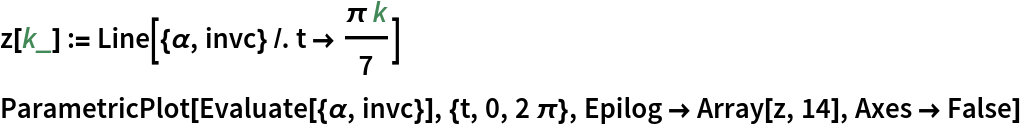Out=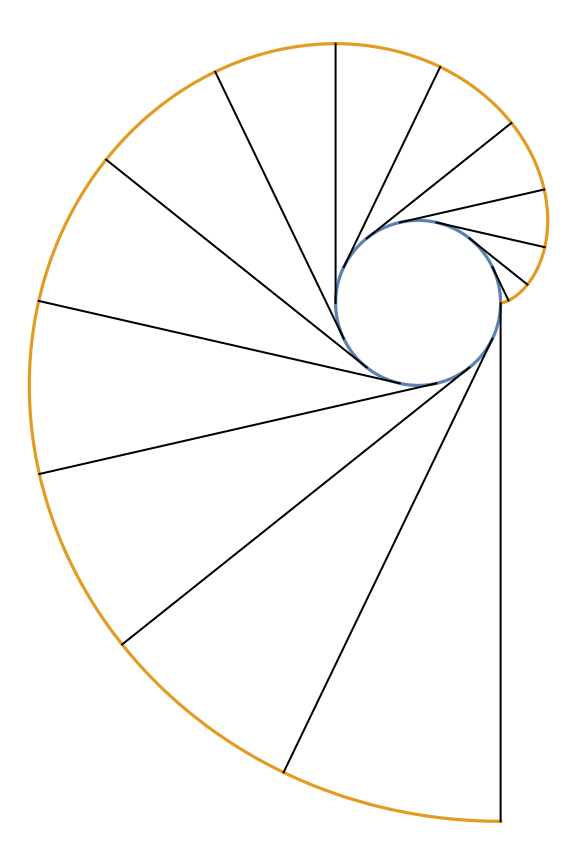Start the involute at π/2:

 In:=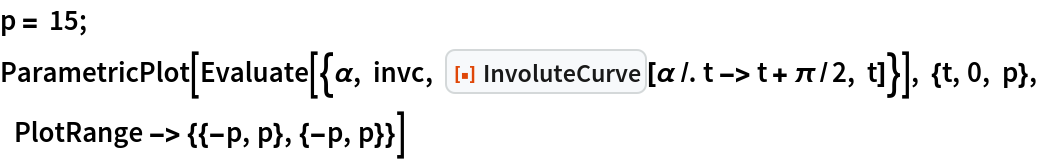Out=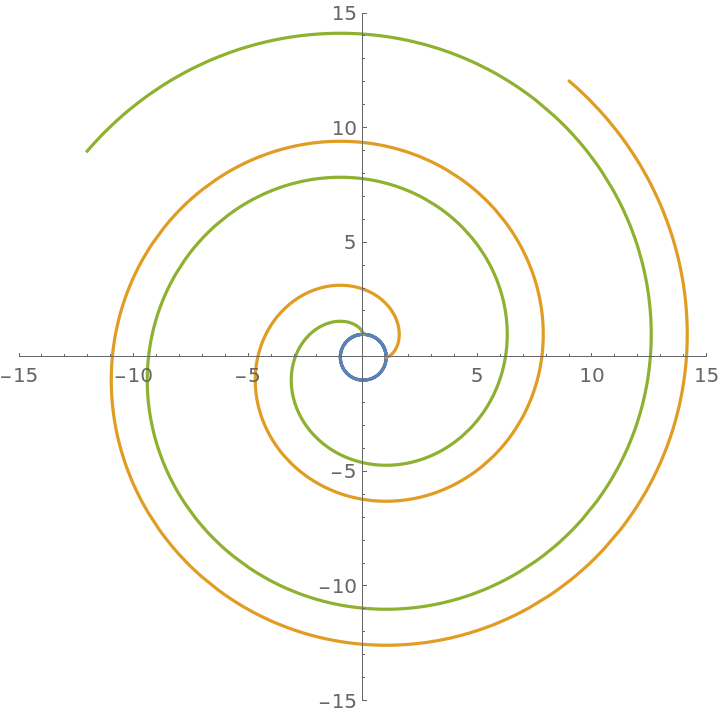Nested involutes of the circle:

 In:=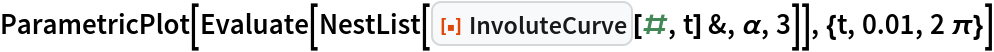Out=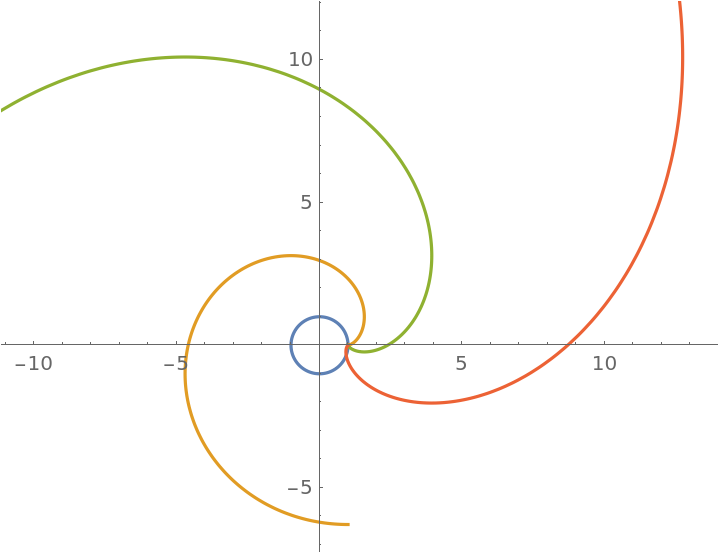Here are some involute plots for several named curves:

 In:=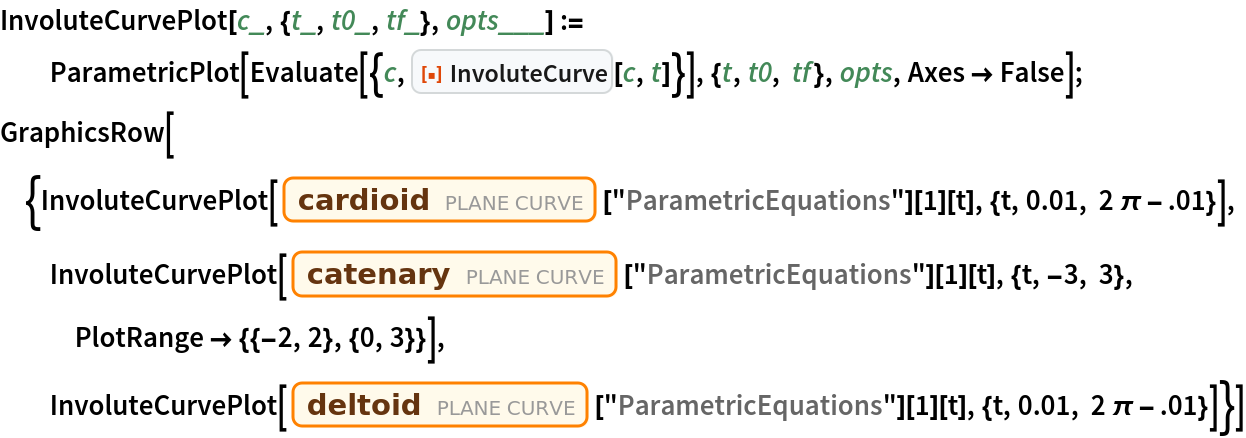Out=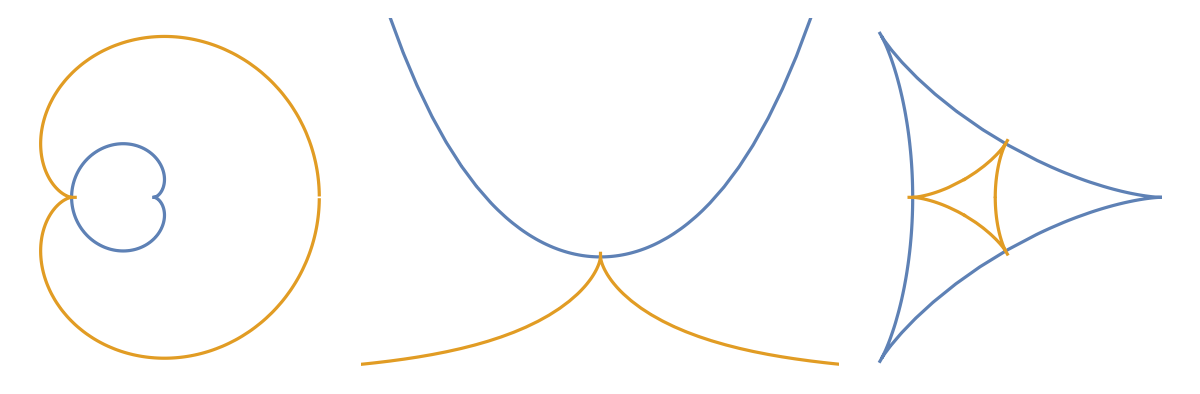### Properties and Relations (1)

The evolute of a curve's involute is the curve itself:

 In:=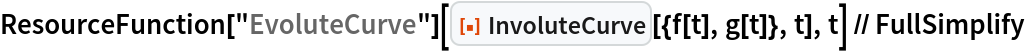Out=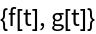### Possible Issues (4)

The eight curve:

 In:=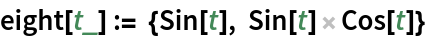The involute curve:

 In:=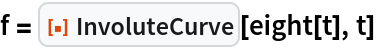Out=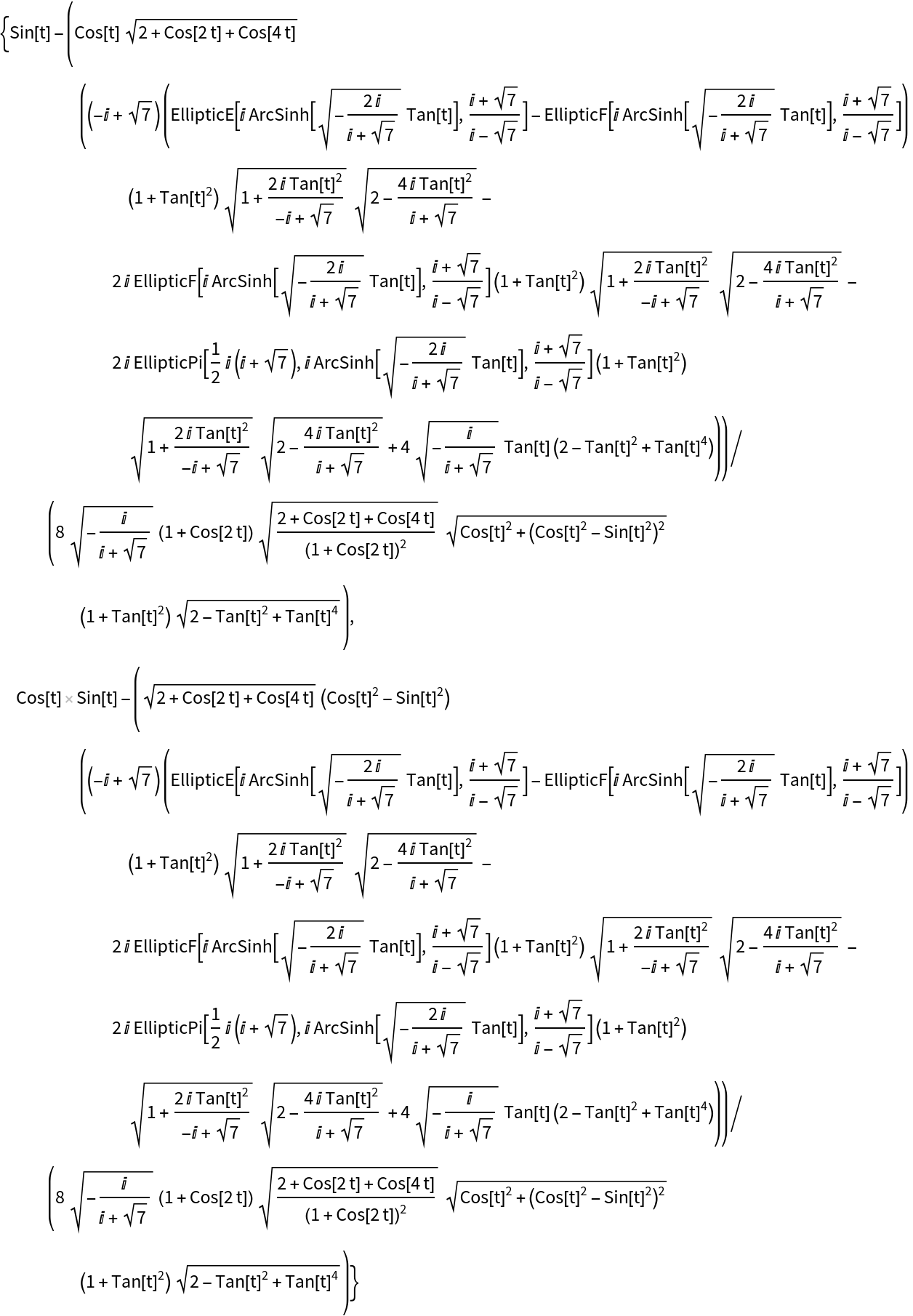The involute curve using the expression shown above gives the same result four times:

 In:=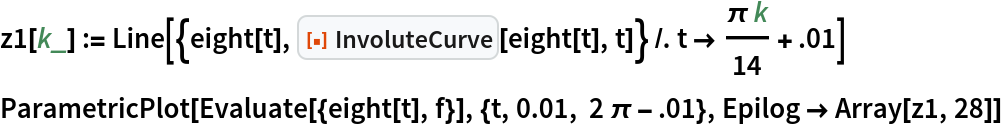Out=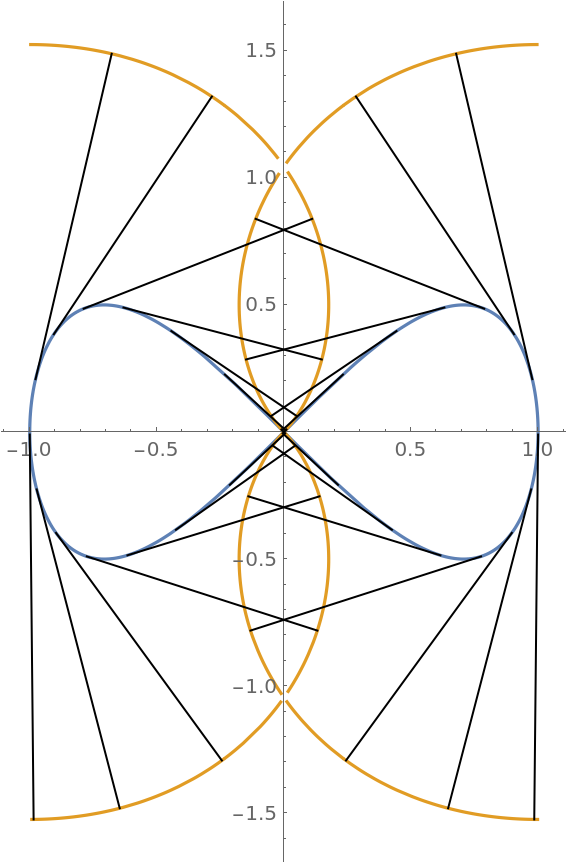Using numerical integration gives the correct result:

 In:=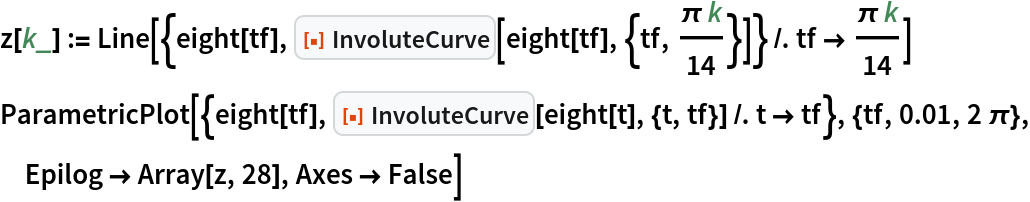Out=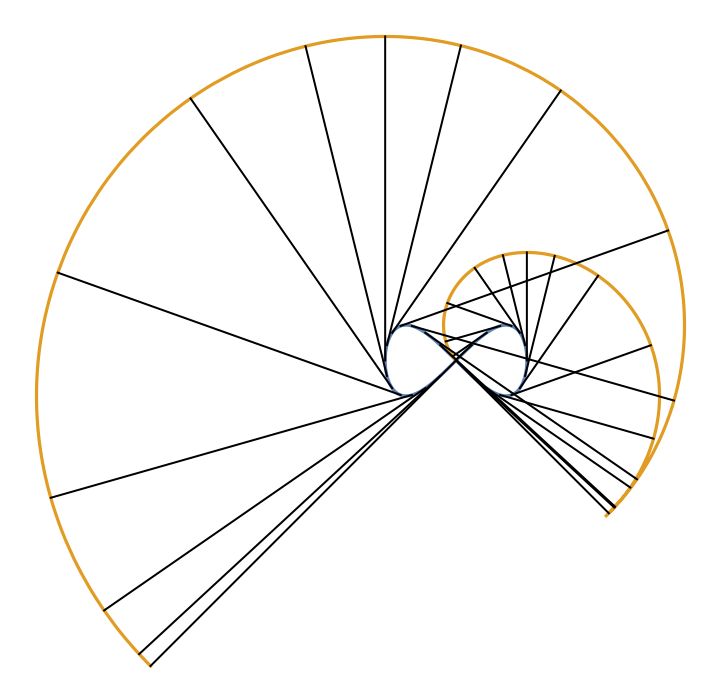Enrique Zeleny

## Version History

• 1.1.0 – 29 March 2021
• 1.0.0 – 16 March 2020